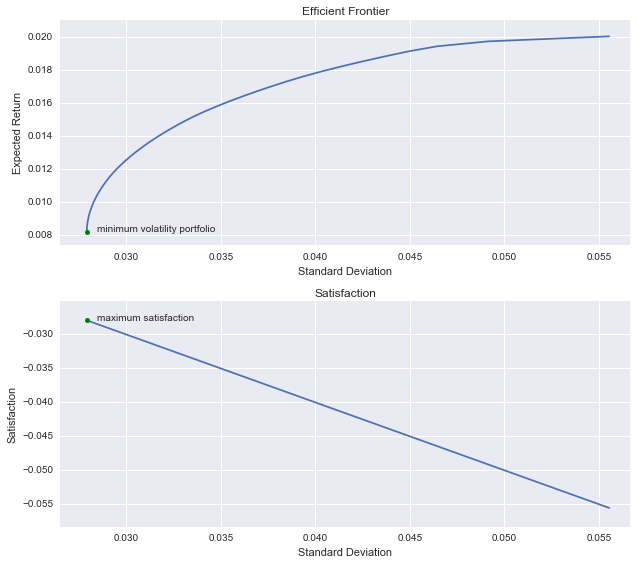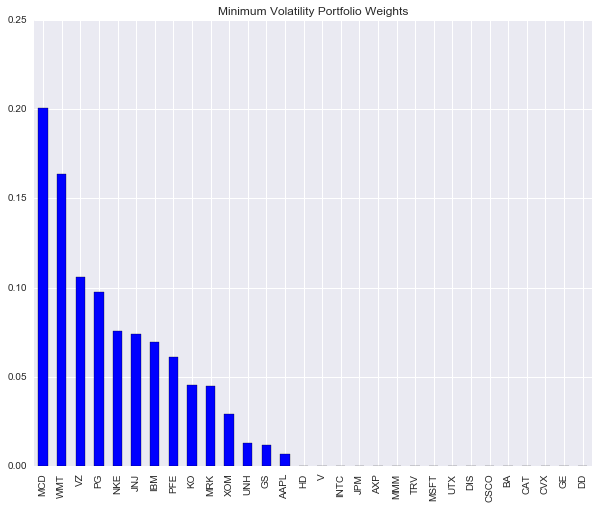# mini-Meucci : Appplying The Checklist - Steps 8-9

"Predicting rain doesn't count. Building arks does."
Warren Buffett, The Oracle of Omaha (born 1930)

In this penultimate leg of the tour we'll be visiting 2 more attractions along Via Meucci, Construction and Execution.

## Construction

Portfolio Construction is another yuge! topic.

In a nutshell, the overall goal is to find optimal holdings that maximize Satisfaction, subject to some type of investment constraints (e.g. on allocation, budget, leverage).

In practice, this type of portfolio optimization problem can be approximately solved (quasi-optimal) via a 2 step mean-variance approach:

1. Calculate the Markowitz mean-variance efficient frontier
2. Choose the efficient allocation that maximises Satisfaction

For more details, read Chapter 6, "Optimizing Allocations", A. Meucci, 2005, Risk and Asset Allocation, (Springer), and go to the ARPM Bootcamp!

### Estimation Risk

One issue to be acutely aware of in this step is estimation risk. In order to calculate the mean-variance efficient frontier, estimates (i.e. expected returns and covariance matrix) of the true unknown distribution are needed.

"...the projected distribution of the P&L that we are optimizing is only an estimate, not the true projected distribution, which is unknown. As it turns out, the optimal portfolio is extremely sensitive to the input estimated distribution..."
The Prayer (Optimization section, former Checklist)

In particular, small changes in the expected returns can lead to extreme weighting changes during the optimization process.

Nonetheless, there are a number of shrinkage techniques that have been developed to address this estimation risk.

For more info, read Chapter 4, "Estimating the distribution of the market invariants", A. Meucci, 2005, Risk and Asset Allocation, (Springer), and Chapter 9, "Optimizing Allocations" A. Meucci, 2005, Risk and Asset Allocation, (Springer).

### Example Python Code

In our toy example with the goal of constructing a low volatility equity portfolio, we'll choose the opposite of volatility as our satisfaction index, as discussed in the previous post.

In this case, the expected return estimates are not actually needed and the optimal portfolio turns out to be the global minimum variance/volatility portfolio. In addition, we apply 2 common constraints, long-only allocations and full investment of the budget (positive weights and weights sum to 1), and use a simple shrinkage technique.

Also, do note that Meucci advises that it is more general to calculate the estimates in \$P&L terms (e.g. for long-short portfolios), but in our simple case, we'll use estimates in % return terms.

In :
%matplotlib inline
import numpy as np
import pandas as pd
import datetime
import math
import matplotlib.pyplot as plt
import seaborn

# Get Yahoo data on 30 DJIA stocks and a few ETFs
tickers = ['MMM','AXP','AAPL','BA','CAT','CVX','CSCO','KO','DD','XOM','GE','GS',
'HD','INTC','IBM','JNJ','JPM','MCD','MRK','MSFT','NKE','PFE','PG',
'TRV','UNH','UTX','VZ','V','WMT','DIS','SPY','DIA','TLT','SHY']
start = datetime.datetime(2008, 4, 1)
end = datetime.datetime(2016, 5, 31)
rawdata = data.DataReader(tickers, 'yahoo', start, end)

# Setup
tau = 21 # investment horizon in days
n_scenarios = len(prices) - tau
n_asset = 30
asset_tickers = tickers[0:30]

# Construction - 2 step mean-variance optimization
# Take shortcut and bypass some of the checklist steps in this toy example since
# returns are invariants, estimation interval = horizon ie can use linear return
# distribution directly as input into mean-variance optimizer

# Projected linear returns to the horizon - historical simulation
asset_rets = np.array(prices.pct_change(tau).ix[tau:, asset_tickers])

# Mean-variance inputs
# Distribution of asset returns at horizon with flexible probabilities
# Time-conditioned flexible probs with exponential decay
half_life = 252 * 2 # half life of 2 years
es_lambda = math.log(2) / half_life
exp_probs = np.exp(-es_lambda * (np.arange(0, n_scenarios)[::-1]))
exp_probs = exp_probs / sum(exp_probs)

# Apply flexible probabilities to asset return scenarios
import rnr_meucci_functions as rnr
mu_pc, sigma2_pc = rnr.fp_mean_cov(asset_rets.T, exp_probs)

# Perform shrinkage to mitigate estimation risk
mu_shrk, cov_shrk = rnr.simple_shrinkage(mu_pc, sigma2_pc)

# Step 1: m-v quadratic optimization for efficient frontier
n_portfolio = 40
weights_pc, rets_pc, vols_pc = rnr.efficient_frontier_qp_rets(n_portfolio,
cov_shrk, mu_shrk)

# Step 2: evaluate satisfaction for all allocations on the frontier
satisfaction_pc = -vols_pc

# Choose the allocation that maximises satisfaction
max_sat_idx = np.asscalar(np.argmax(satisfaction_pc))
max_sat = satisfaction_pc[max_sat_idx]
max_sat_weights = weights_pc[max_sat_idx, :]
print('Optimal portfolio is minimum volatility portfolio with satisfaction\
index = {:.2}'.format(max_sat))

# Plot charts
import matplotlib.gridspec as gridspec
fig = plt.figure(figsize=(9, 8))
fig.hold(True)
gs = gridspec.GridSpec(2, 1)
ax.plot(vols_pc, rets_pc)
ax.set_xlim(vols_pc*0.95, vols_pc[-1]*1.02)
ax.set_ylim(min(rets_pc)*0.9, max(rets_pc)*1.05)
ax.set_xlabel('Standard Deviation')
ax.set_ylabel('Expected Return')
ax.set_title("Efficient Frontier")
ax.plot(vols_pc, rets_pc, 'g.', markersize=10.0)
ax.text(vols_pc*1.02, rets_pc, 'minimum volatility portfolio',
fontsize=10)

ax2.plot(vols_pc, satisfaction_pc)
ax2.set_xlim(vols_pc*0.95, vols_pc[-1]*1.02)
ax2.set_ylim(min(satisfaction_pc)*1.05, max(satisfaction_pc)*0.9)
ax2.set_xlabel('Standard Deviation')
ax2.set_ylabel('Satisfaction')
ax2.set_title("Satisfaction")
ax2.plot(vols_pc[max_sat_idx], max(satisfaction_pc), 'g.', markersize=10.0)
ax2.text(vols_pc[max_sat_idx]*1.02, max(satisfaction_pc), 'maximum satisfaction',
fontsize=10)
plt.tight_layout()
plt.show()

# Plot minimum volatility portfolio weights
pd.DataFrame(weights_pc[0,:], index=asset_tickers, columns=['w']).sort_values('w', \
ascending=False).plot(kind='bar', title='Minimum Volatility Portfolio Weights', \
legend=None, figsize=(10, 8))
plt.show()

Optimal portfolio is minimum volatility portfolio with satisfaction index = -0.028## Execution

The goal of this step is to achieve the optimal (target) allocation by rebalancing the current allocation.

To optimize the execution strategy and achieve the optimal allocation, the following steps are applied recursively:

1. Order scheduling
2. Order placement
3. Order routing (optional)

Do note that execution/transaction costs including fees and slippage/shortfall can have a material impact on portfolio performance.
As for a python code example, I'll include this in the next post on Dynamic Allocation when backtesting with the Quantopian open source Zipline package.

Final stop on the tour is Dynamic Allocation and Ex-post Performance Analysis...

P.S. If you do sign-up for the Bootcamp, please let the good folks at ARPM know you learnt about it at returnandrisk.com!

## References to Attilio Meucci's Work

1. The Checklist slides
2. The Prayer (former Checklist)
3. Chapter 6, "Optimizing Allocations", A. Meucci, 2005, Risk and Asset Allocation, (Springer)
4. Chapter 4, "Estimating the distribution of the market invariants", A. Meucci, 2005, Risk and Asset Allocation, (Springer)
5. Chapter 9, "Optimizing Allocations", A. Meucci, 2005, Risk and Asset Allocation, (Springer)